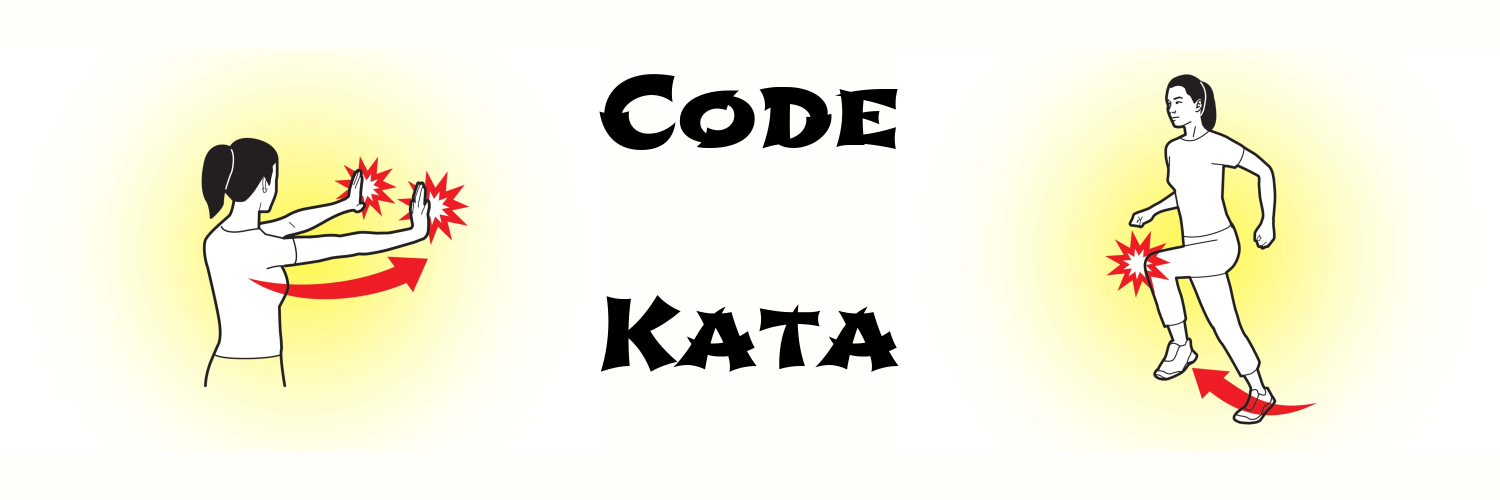# programming kata

## Programming Kata Day 4Problem Implement take-nth. Solutions Solution 1 (defn my-take-nth [n col] (loop [i 0 result []] (if (= i (count col)) result (if (= 0 (mod i n)) (recur (inc i) (conj result (nth col i))) (recur (inc i) result))))) solution 2 (defn my-take-nth2 [n col] (->> col (map-indexed (fn [i x] [i x])) (filter (fn [[i x]] (= 0 (mod i 2)))) (mapv (fn [[i x]] x))))

## programming kata day 3Problem Trolls are attacking your comment section! A common way to deal with this situation is to remove all of the vowels from the trolls' comments, neutralizing the threat. Your task is to write a function that takes a string and return a new string with all vowels removed. For example, the string “This website is for losers LOL!” would become “Ths wbst s fr lsrs LL!”. Solution Solution 1 (defn disemvowel [string] (reduce (fn [result next] (if (#{\A \E \I \O \U \a \e \i \o \u} next) result (str result next))) "" string)) Solution 2 (defn disemvowel [string] (apply str (remove (set "AEIOUaeiou") string)))

## Programming Kata Day 1